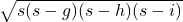## In ΔGHI, h = 10 cm, ∠G=152° and ∠H=7°. Find the area of ΔGHI, to the nearest square centimeter.

Question

In ΔGHI, h = 10 cm, ∠G=152° and ∠H=7°. Find the area of ΔGHI, to the nearest square centimeter.

in progress 0
6 months 2021-07-23T09:01:11+00:00 1 Answers 19 views 0

## Answers ( )

Step-by-step explanation:

I = 180-152-7 = 21°

Law of Sines:

g = h·sinG/sinH ≅ 38.5 cm

i = h·sinI/sinH = 29.4 cm

Use Heron’s Formula to calculate the area from the lengths of the sides.

semi-perimeter s = (g+h+i)/2 ≅ 39.0 cm

area =≅ 69 cm²